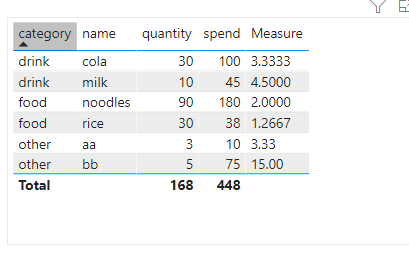cancel
Showing results for
Did you mean:Anonymous
Not applicable

## rounding decimals

i have a dax formula that calculate a cost per unit(spending divided by quantity). but when filter is applied for certain category such as , if category for food or beverages is selected, the cost per unit calculated by the dax formula should be showing 4 decimals place, if other category is selected it should show 2 decimals places. how should the DAX formula be written?

1 ACCEPTED SOLUTIONSuper User

Hi @Anonymous

You might have to use calculation groups for this:

https://www.sqlbi.com/articles/controlling-format-strings-in-calculation-groups/Please accept the solution when done and consider giving a thumbs up if posts are helpful.  Contact me privately for support with any larger-scale BI needs, tutoring, etc.

3 REPLIES 3Community Support

Hi @Anonymous ,

Create a measure.

``````Measure = var price_each=DIVIDE(SELECTEDVALUE('Table'[spend]),SELECTEDVALUE('Table'[quantity]))
var result= IF(SELECTEDVALUE('Table'[category])in {"food","drink"},FORMAT(price_each,"0.0000"),FORMAT(price_each,"0.00"))
return result``````Best Regards

Community Support Team _ Polly

If this post helps, then please consider Accept it as the solution to help the other members find it more quickly.Super User

Hi @Anonymous

You might have to use calculation groups for this:

https://www.sqlbi.com/articles/controlling-format-strings-in-calculation-groups/Please accept the solution when done and consider giving a thumbs up if posts are helpful.  Contact me privately for support with any larger-scale BI needs, tutoring, etc.Super User

@Anonymous , Actually Might have to use format, but that might make it text

if(max(table[Category] in {"food","beverages"}, Format([Measure], #,###.0000) , Format([Measure], #,###.00)  )